• ### Browse All Lessons

##### Assign Lesson

Help Teaching subscribers can assign lessons to their students to review online!

 Tweet# Speed

This lesson aligns with Next Generation Science Standards (NGSS) PS2.A

Introduction
Distance is the measurement of how far an object travels. For example, a car driving on a highway moves 30 kilometers. If a car covers a distance of 30 kilometers in a certain amount of time. Then it is easy to calculate the speed of a car. Speed is actually a type of rate. A rate tells the quantity of something that changes in one unit of time. Speed plays a significant role in describing the motion of an object. In this article, we will learn about speed, how to calculate the speed of an object, and types of speed.

Speed
The speed of an object can be defined as “the time rate at which an object is moving along a path.”  In other words, speed is the rate of change of position of a body in any direction. For instance, a vehicle covers a 10-kilometer distance in 30 minutes. This is the speed of the vehicle shown on the speedometer. Speed measures the ratio of the distance traveled by an object to the time in which the distance was covered. Speed is a scalar quantity that has only magnitude.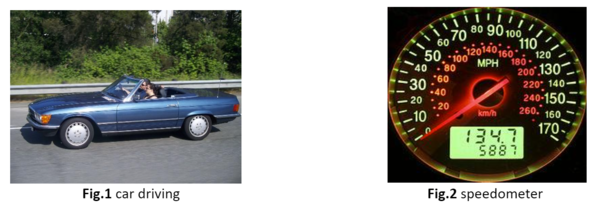Calculating Speed
To calculate the speed of an object, divide the distance traveled by the amount of time it takes to cover that distance. This relationship can be written as: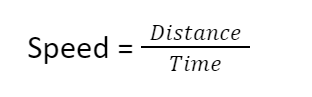If the distance is measured in meters, and time is measured in seconds, then speed is expressed in meters per second or m/s. If the distance is measured in a kilometer, and time in hours, then speed can also be expressed in kilometers per hour or km/h. For example, a car driving on a highway covers 30 kilometers in one hour. The speed of a car will be 30 km/h.

Another example of a person who starts at point A walks 10 metes to point B, then walks another 5 metes to point C. The total distance is 15 meters. If he covers that distance in 90 seconds, then the speed will be 0.16 m/s.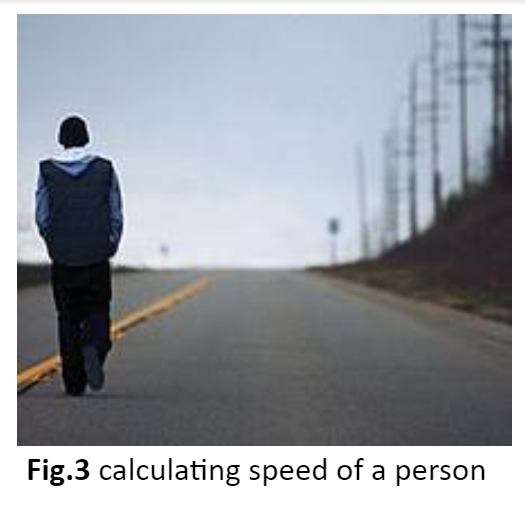Types of Speed
Four types of speed are discussed below.

Uniform Speed
When an object covers an equal distance in an equal interval of time, then the object is said to be in uniform speed. For example, a cyclist is traveling on a straight track, he covers 5 cm every second. Then the cyclist is said to be moving with a uniform speed.

Variable Speed
When an object covers a different distance in an equal interval of time, then the object is said to be in variable speed. For example, a cyclist is said to be moving with variable speed if he covers 5 km in the first 10 minutes, 10 km in the second 10 minutes, and 12 km in the next 10 minutes, and so on.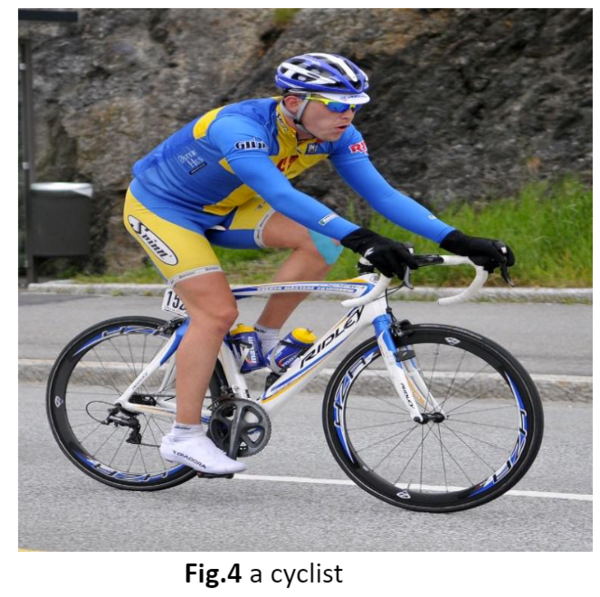Average Speed
The speed of the moving objects is not constant. The cars shown in the figure change their speed during the race. They might move with constant speed on the straight track but move more slowly as they climb the bridge. Then they might move quickly as they come down the bridge. At some point, they may stop to fix their brakes. The speed of the car was not constant but average throughout the race.

Average speed can be calculated by the ratio of the total distance traveled by the object to the time taken by the object. Suppose a car travels 20 km during the first hour. Then the car travels 40 km during the next 2 hours.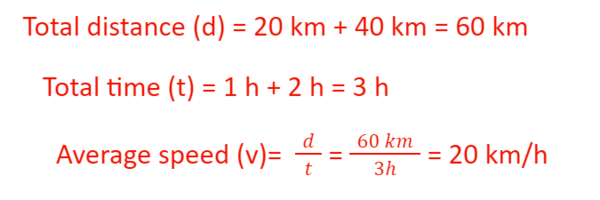The average speed of the car is 20 km/h. if two quantities are known, the third quantity can be calculated easily.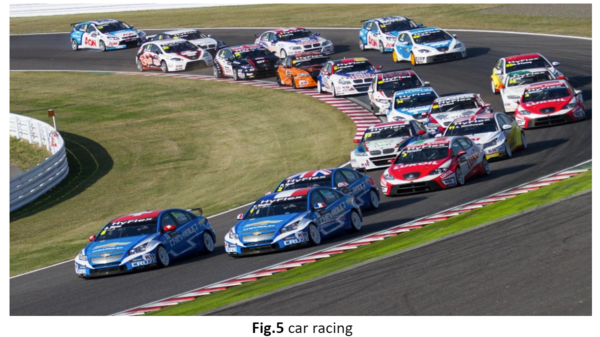Instantaneous Speed
The rate at which an object is moving at any instant of time is known as instantaneous speed. It is not only important to know the average speed, but it is also useful to know the instantaneous speed of a moving object.

Summary
• Speed is the rate of change of position of an object without considering its direction. It is a scalar quantity.
• Speed is measured by the ratio of the distance traveled by an object to the time in which that distance was covered.
• When an object covers an equal distance in an equal interval of time, then the speed of the object is said to be in uniform speed.
• If an object covers a different distance in an equal interval of time, that kind of speed is known as variable speed.

Related Worksheets: